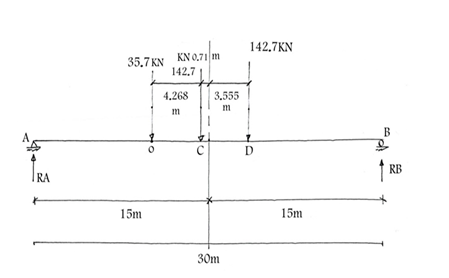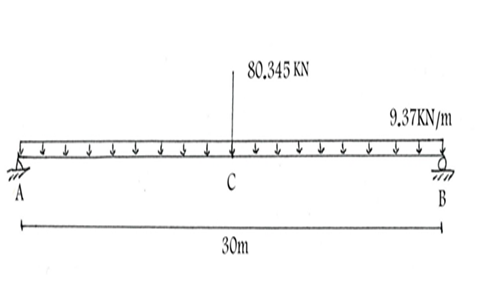##### MOJ
###### eISSN: 2572-8520# Civil EngineeringReview Article Volume 4 Issue 2

# Multiplication bending moment factor for AASHTO live Loads adopted in Jordan

#### Samih Qaqish function clickButton(){ var name=document.getElementById('name').value; var descr=document.getElementById('descr').value; var unCopyslNo=document.getElementById('unCopyslNo').value; document.getElementById("mydiv").style.display = "none"; $.ajax({ type:"post", url:"https://medcraveonline.com/captchaCode/server_action", data: { 'name' :name, 'descr' :descr, 'unCopyslNo': unCopyslNo }, cache:false, success: function (html) { //alert('Data Send');$('#msg').html(html); } }); return false; } Verify Captcha Regret for the inconvenience: we are taking measures to prevent fraudulent form submissions by extractors and page crawlers. Please type the correct Captcha word to see email ID.function refreshPage(){ $("#mydiv").load(location.href + " #mydiv"); }$(document).ready(function () { //Disable cut copy paste $('#msg').bind('cut copy paste', function (e) { e.preventDefault(); }); //Disable mouse right click$("#msg").on("contextmenu",function(e){ return false; }); }); .noselect { -webkit-touch-callout: none; /* iOS Safari */ -webkit-user-select: none; /* Safari */ -khtml-user-select: none; /* Konqueror HTML */ -moz-user-select: none; /* Firefox */ -ms-user-select: none; /* Internet Explorer/Edge */ user-select: none; /* Non-prefixed version, currently supported by Chrome and Opera */ cursor: none; }

Ex-Dean Faculty of Engineering, University of Jordan, Jordan

Correspondence: Samih Qaqish, Ex-Dean Faculty of Engineering, University of Jordan, Amman, Jordan, Tel 962-777-427-511

Received: November 20, 2017 | Published: April 23, 2018

Citation: Qaqish S. Multiplication bending moment factor for AASHTO live Loads adopted in Jordan. MOJ Civil Eng. 2018;4(2):104-107. DOI: 10.15406/mojce.2018.04.00105

# Abstract

This result will be used for the design of highway bridges in Jordan, and it will be a reference number for the Arab Countries also for such subject.

# Introduction

The live loads of the AASHTO specifications (LFD) consist of standards trucks or off– lane loads as shown in Figure 1. While the live loads of the AASHTO specifications (2) LRFD is HL–93 which consists of truck loading and distributed load of 9.3 KN/m as shown in Figure 2. The impact factor for LFD is calculated from:

While the Dynamic load allowance is considered 33% for LRFD.

# Structural idealization

Figure 3 shows the plan of bridge, section A–A, and section B–B. The span of the bridges is 30m with two lanes, one lane in each direction. Box Type Bridge is considered with 7.8 m total width and 2.2 m total depth.

Moment due to HS20–44

Maximum positive moment (Figure 4).Figure 4 Truck Hs 20–44 location for Max moment.

$\text{ΣMB=0}\text{.0}$

${\text{R}}_{\text{A}}×\text{30=35}\text{.7}×\text{19}\text{.978}+\text{142}\text{.7}×\text{142}\text{.7}×\text{11}\text{.445}$
${\text{R}}_{\text{A}}\text{=(713}\text{.22}+\text{2241}\text{.817}+\text{1633}\text{.2)/30}$
${\text{R}}_{\text{A}}\text{=152}\text{.94KN}$
${\text{R}}_{\text{B}}\text{=168}\text{.16KN}$

Max. moment at C

${\text{M}}_{\text{C}}=\text{152}\text{.94}×\text{14}\text{.29}-\text{35}\text{.7}×\text{4}\text{.268}$
$\text{\hspace{0.17em}}\text{ }=\text{2185}\text{.5}-\text{152}\text{.4}=\text{2033}\text{.1KN}\text{.m}$

Impact Factor      = $\frac{\text{50}}{\text{L}+\text{125}}$=$\frac{\text{50}}{\text{3}\text{.28}×\text{30}+\text{125}}$= 22.4%

Total live load bending moment = 2 x 2033.1 x 1.224 =4977.029 kN.m

Figure 5 shows the maximum moment due to equivalent uniform loadings of HS 20–44.Figure 5 Equivalent uniform load for HS 20–44.

Bending moment at C = $\frac{\text{W}{\text{L}}^{\text{2}}}{\text{8}}+\frac{\text{PL}}{\text{4}}$$\text{=}\frac{\text{9}\text{.37}×\text{3}{\text{0}}^{\text{2}}}{\text{8}}+\frac{\text{80}\text{.345}×\text{30}}{\text{4}}$$\text{=1054}\text{.125}+\text{602}\text{.59}$$\text{=165}\text{.7125}$

Total live equivalent bending moment = 2 x 1656.7125 x 1.224 = 4055.63 KN.m

So the bending moment due to the Truck Loading HS 20–44 governs and it's value 4977.029 KN.m.

LRFD

Figure 6 shows the location of HL– 93 Truck loading to give maximum moment at point O.Figure 6 Truck HL–93 location for max. moment.

${\text{R}}_{\text{A}}×\text{30}=\text{35}×\text{20}\text{.01}+\text{145}×\text{15}\text{.71}+\text{145}×\text{11}\text{.41}$$=\text{700}\text{.35}+\text{2277}\text{.95}+\text{1654}\text{.45}$

${\text{R}}_{\text{A}}=\text{154}\text{.425K}$

Bending moment due to distributed loadTotal live bending moment

Where 33% is the impact factor, and it is calculated for two lanes.

Bending moment due to own weight of the bridge

LFD

B. M. due to own weight of the bridge

$\frac{\text{97}\text{.6}×\text{3}{\text{0}}^{\text{2}}}{\text{8}}\text{=10980KN}\text{.m}$

B.M. due to wearing surface

$\frac{\text{7}\text{.56}×\text{3}{\text{0}}^{\text{2}}}{\text{8}}\text{=850}\text{.5KN}\text{.m}$

Total B.M=1.3

LRFD

So the factor to make the bending moment due to LFD equal to LRFD is:

So the live loads for LRFD should be multiplied by 1.33 to make the bending moment due to 1.8 HS 20–44 equal to the moment due to LRFD loadings.

Finite element analysis was carried out for the bridge using CSi bridge software (16), and the results are:

Total B. M. (LFD)                36332 KN. m.
Total B. M. (LRFD)             27995 KN. m.

$\text{Factor=}\frac{\text{36332}}{\text{27995}}\text{=1}\text{.3}$

So it can be noticed that the factor from the structural calculations and the Csibridge is almost identical, and it is recommended to use 1.35 as a factor.

# Conclusion

The live loads for LRFD designated as HL–93 which consist of design truck or design tandem and design lane load should be multiplied by 1.35 to encounter the unexpected traffic loading in Jordan and to be equivalent to 1.8 HS 20–44 LFD which is adopted in design bridges in Jordan to get the same bending moment for LFD and LRFD. This result will be used in Jordan for designing bridges after will be adopted in the Ministry of Public works and Housing.

None.

# Conflicts of interest

The authors declare no conflict of interest.